How to Learn in 24 Hours?The Rapid Learning Movie

 Need Help? M-F: 9am-5pm(PST): Toll-Free: (877) RAPID-10 US Direct: (714) 692-2900 Int'l: 001-714-692-2900 24/7 Online Technical Support: The Rapid Support Center Secure Online Order:Need Proof? Testimonials by Our Users

 Rapid Learning Courses: MCAT in 24 Hours (2015-16) USMLE in 24 Hours (Boards) Chemistry in 24 Hours Biology in 24 Hours Physics in 24 Hours Mathematics in 24 Hours Psychology in 24 Hours SAT in 24 Hours ACT in 24 Hours AP in 24 Hours CLEP in 24 Hours DAT in 24 Hours (Dental) OAT in 24 Hours (Optometry) PCAT in 24 Hours (Pharmacy) Nursing Entrance Exams Certification in 24 Hours eBook - Survival Kits Audiobooks (MP3)

 Tell-A-Friend: Have friends taking science and math courses too? Tell them about our rapid learning system.Home »  Mathematics »  Pre-Calculus

Law of Sines and Cosines

 Topic Review on "Title": Law of Sines: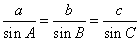= side of triangle ABC divide by the sine of angle opposite side Law of Cosines: For a triangle ABC to find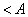, b and c we use the formula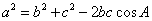. For a triangle ABC to find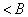, a and c then we use the formula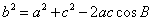. For a triangle ABC to find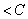, a and b then we use the formula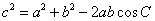.

Rapid Study Kit for "Title":
 Flash Movie Flash Game Flash Card Core Concept Tutorial Problem Solving Drill Review Cheat Sheet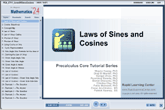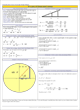"Title" Tutorial Summary : The law of sines and cosines are important to know so solutions to trigonometry application problems can be found. Knowing which rule to use in the law of sines and cosines problems is important to achieve a good solution to a law of sines and cosines problem.  Scenarios are given where some angles or sides of a triangle are not known so the law of sines and cosines are used in examples. Acronyms are giving for the different cases of the law of sines and the various cases of the law of cosines.

 Tutorial Features: Specific Tutorial Features: • Diagrams showing various law of sines and cosines scenarios. • Illustrations showing all aspects of the law of sines and cosines. Series Features: • Concept map showing inter-connections of new concepts in this tutorial and those previously introduced. • Definition slides introduce terms as they are needed. • Visual representation of concepts • Animated examples—worked out step by step • A concise summary is given at the conclusion of the tutorial.

 "Title" Topic List: Law of Sines Cycle representation Side-Angle Side (SAS) Angle-Side-Angle (ASA) Side-Side-Angle (SSA)Law of Cosines Side-Angle-Side (SAS) Side-Side-Side (SSS)

See all 24 lessons in Pre-Calculus, including concept tutorials, problem drills and cheat sheets:
Teach Yourself Pre-Calculus Visually in 24 Hours